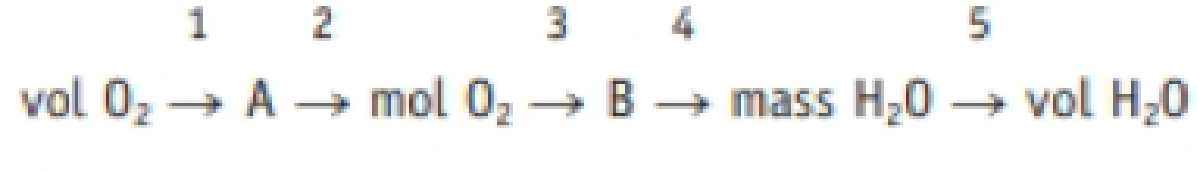Chapter 4.9, Problem 4.2ACP

Chapter
Section
Textbook Problem

Identify the factor labeled 3 in this strategy map. (a) 1 mol O2/1 mol H2O (b) 1 mol O2/2 mol H2O (c) 1 mol H2O/1 mol O2 (d) 2 mot H2O/1 mol O2Interpretation Introduction

Interpretation:

The factor labeled 3 in the given strategy map has to be identified.

Concept introduction:

• The relation between the number of moles and mass of the substance is ,

Numberofmole=MassingramMolarmass

Massingramofthesubstance=Numberofmole×Molarmass

• The molar mass of an element or compound is the mass in grams of 1 mole of that substance, and it is expressed in the unit of grams per mole (g/mol).
• For chemical reaction balanced chemical reaction equation written in accordance with the Law of conservation of mass.
• Law of conservation of mass states that for a reaction total mass of the reactant and product must be equal.
• Stoichiometric factor is a relationship between reactant and product which is obtained from the balanced chemical equation for a particular reaction.
Explanation

Reason for the correct option:

The given strategy map is,

VolO21A2molO23B4MassofH2O5VolH2O

Here A is the mass of O2 and B is the amount of H2O.

The balanced chemical equation for the reaction involving hydrogen and oxygen as a reactant is,

2H2+O22H2O

From the amount of O2 number of moles of H2O(B) can be calculated

Still sussing out bartleby?

Check out a sample textbook solution.

See a sample solution

The Solution to Your Study Problems

Bartleby provides explanations to thousands of textbook problems written by our experts, many with advanced degrees!

Get Started